So far, we identified the countries you should focus on at the release of your next hit, the features you should consider, the nationality that could help your song hit the top charts, and the kind of music you should play.

In this article, we are going to focus mainly on the lyrics you should write.

## The data

Our first step was to fetch the lyrics of all top 200 songs of the Spotify charts. To do so, we use the Genius.com API. We found a great library that does not require a Redirect URI called: `lyricsgenius`.

The tracks we want to dowload the datas from are the following :

``````tracks = pd.read_csv('Tracks.csv')
tracks = tracks.sort_values('Streams', ascending = False)
``````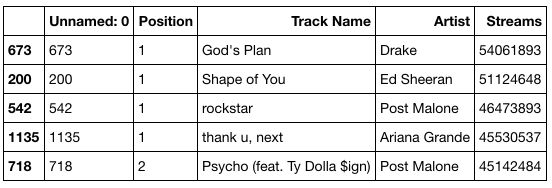Then, using `lyricsgenius` :

``````api = genius.Genius('VGxZYl4kHnoBcj_hMiUA0DtweOQvySa8c7hi_fvyqbKd__3or_Lkn75yCG6_immb')

i = 0
for track in zip(tracks['Track Name'], tracks['Artist']) :
try :
song = api.search_song(str(track), str(track))
song.save_lyrics('***/Hackathon/New_songs/' + str(track + str(i)))
except :
pass
i = i + 1
``````

This will write the songs lyrics in separate .txt files in a new folder. To treat them at a single file :

``````files = sorted(glob(op.join('***/Hackathon/New_songs/', '*.txt')))
songs = [open(f).read() for f in files]
``````

We proceed to a quick text cleaning to remove line jumps, backslashes and expressions in brackets :

``````for i in range(0, len(songs)) :
songs[i] = songs[i].replace("\n", " ").replace("\'", " ")
songs[i] = re.sub(r"\[(.*?)\]", " ", songs[i])
``````

Then we remove stop words :

``````cachedStopWords = stopwords.words("english")

words = []
filtered = []

for i in range(0, len(songs)) :
words.append(re.split("(?:(?:[^a-zA-Z]+')|(?:'[^a-zA-Z]+))|(?:[^a-zA-Z']+)", songs[i]))
filtered.append(' '.join([word for word in songs[i].split() if word not in cachedStopWords]))
``````

The `words` variable is a list of list that contains all individual words of each song. We made here a pretty big assumption that all the lyrics are in english.

Then, the variable we are interested in are typically:

• how many words are used on average?
• how many times is each word repeated on average?
• and how many words are left when we remove stop words and repeated words?
``````voc = []
voc_unique = []

for i in range(0, len(songs)) :
voc.append(len(words[i]))
voc_unique.append(len(filtered[i].split()))
``````

## How many words on average?

``````plt.figure(figsize=(12,5))
plt.hist(np.array(voc), bins=40)
plt.title('Number of words in a top 2018 song')
plt.show()
``````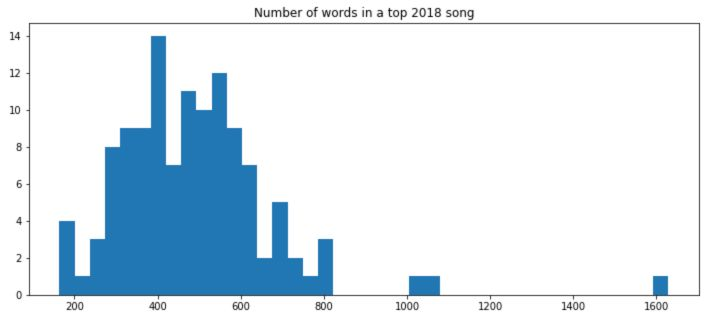On average, a hit song is 490 words long.

## How many time should you repeat each word?

``````length = [len(set(word)) for word in words]
round(np.array(length).mean(),2)
``````

On average, only 161 distinct words are used, which means an average of each words being repeated 3 times.

``````plt.figure(figsize=(12,5))
plt.hist(np.array(length), bins=40)
plt.title('Number of unique words in a top 2018 song')
plt.show()
``````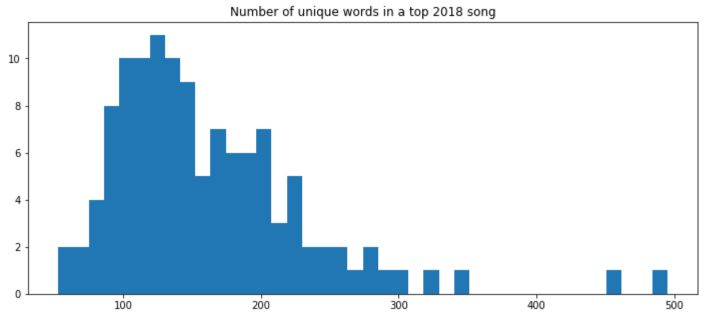## And if we remove stop words?

Removing stop words dramatically reduces the number of words left.

``````length_fil = [len(set(word)) for word in filtered]
round(np.array(length_fil).mean(),2)
``````

Only 45.5 words left on average.

``````plt.figure(figsize=(12,5))
plt.hist(np.array(length_fil), bins=40)
plt.title('Number of unique words in a top 2018 song, without stopwords')
plt.show()
``````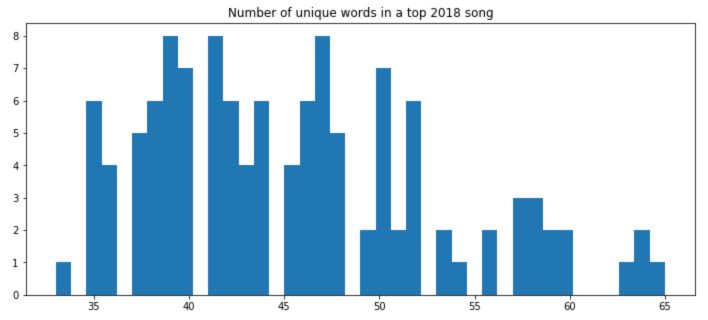## What words should you use?

For a graphical view on this question, we decided to use a WordCloud representation.

``````word_cloud = list(itertools.chain.from_iterable(words))

str1 = ' '.join(word_cloud)
stopwords = set(STOPWORDS)

wordcloud = WordCloud(stopwords=stopwords, background_color="white").generate(str(str1))

plt.figure(figsize=(15,8))
plt.imshow(wordcloud, interpolation='bilinear')
plt.axis("off")
plt.show()
``````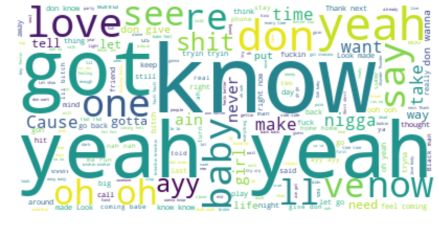## Should the lyrics be positive?

For this question, we use an NLTK pre-trained model on the IMDb dataset. This pre-trained model is trained to identify positive and negative comments for movies reviews.

``````filename = 'model_sentiment_analysis.sav'

print(result.mean())
``````

The average positivity of the lyrics is 0.325. On average, among our data set, only 32% of the songs express a positive feeling.

## Going deeper in the feelings…

We also used another pre-trained model on this one to detect a few sentiments: Angry, Sad, Happy and Relax.

``````filename = 'sentiment_model.sav'